# IBPS PO Prelims Reasoning Ability Questions 2021 (Day-02)

Dear Aspirants, Our IBPS Guide team is providing new series of Reasoning Questions for IBPS PO Prelims 2021 so the aspirants can practice it on a daily basis. These questions are framed by our skilled experts after understanding your needs thoroughly. Aspirants can practice these new series questions daily to familiarize with the exact exam pattern and make your preparation effective.

Start Quiz

Linear arrangement

Direction (1-5): Study the following information carefully and answer the below questions.

Eight persons S, T, U, V, W, X, Y, and Z are sitting in a straight row facing north but not necessarily in the same order. Consecutive alphabetical name of the persons doesn’t sit adjacent to each other.

Y sits third from one of the ends. Only one person sits between W and Y.Only one person sits between Y and S.Z sits to the immediate right of the one who sits second to the left of X.Neither X nor Z is sitting at an extreme end. The number of persons sits to the left of T is one less than the number of persons sit to the right of Z. V sits adjacent to neither W nor X.

1) Who among the following person sits to the immediate left of the one who sits second to the right of V?

A.S

B.X

C.W

D.T

E.None of these

2) Who sits second to the left of V?

A.T

B.Y

C.W

D.S

E.Z

3) If W is related to Y and V is related to Z in a certain way. Then, who among the following is related to X?

A.S

B.T

C.Y

D.Z

E.None of the above

4) Four of the five among the following are similar in such a way to forms a group, who among the following doesn’t belong to that group?

A.T

B.Y

C.V

D.S

E.U

5) What is the position of V with respect to U?

A.Second to the right

B.Second to the left

C.Third to the left

D.Fourth to the left

E.None of the above

Order and ranking

Direction (6-8): Study the following information carefully and answer the below questions.

There are six persons- P, Q, R, S, T, and U belong to a different height. P is taller than Q. U is taller than S. Three persons are between Q and P. The number of persons taller than S is the same as shorter than R. U is neither the tallest nor the shortest one. The second tallest person’s height is 165cm.

6) What may be the height of P?

A.162

B.160

C.164

D.168

E.None

7) How many persons are taller than S?

A.One

B.Two

C.Three

D.Four

E.Can’t be determined

8) If the sum of the height of U and S is 325, and S+R=315 then what is the height of R?

A.160cm

B.145cm

C.155cm

D.165cm

E.None of the above

Misc

9) If in the number 563812479354, the digits at even positions (from the left end) are dropped and the remaining digits are arranged in ascending order from the left end, then which of the following number is second from the right end?

A.1

B.4

C.5

D.3

E.9

10) If a meaningful word can be formed by using T, S, H, and A using each letter only once then what is the second letter from the left end of the newly formed word? Mark Y as your answer if more than one word is formed. Mark Z if no meaningful word can be formed.

A.A

B.P

C.H

D.Y

E.Z

Directions (1-5) :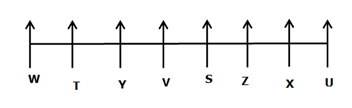Y sits third from one of the ends.

Only one person sits between W and Y.

Only one person sits between Y and S.

From the above condition, there are four possibilities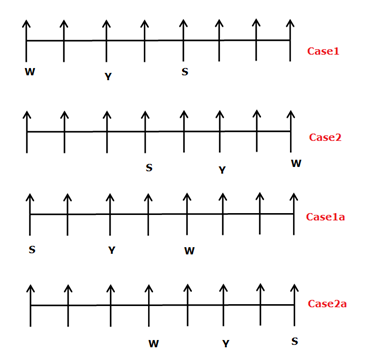Z sits to the immediate right of the one who sits second to the left of X.

Neither X nor Z is sitting at an extreme end.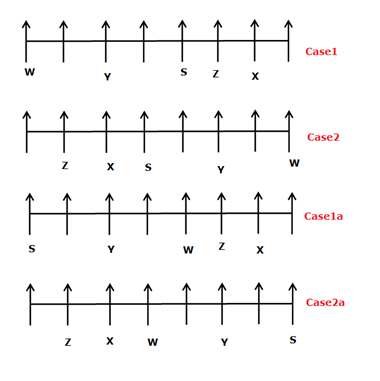The number of people sit to the left of T is one less than the number of people sit to the right of Z.

From the above condition, case2 and case2a are dropped.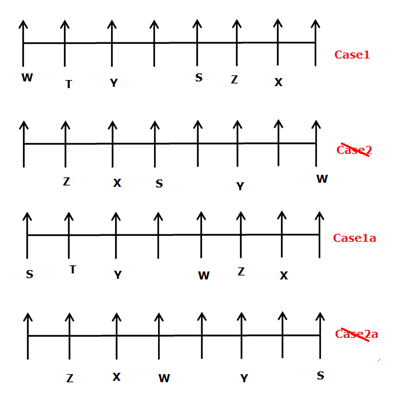V sits adjacent to neither W nor X.

From the above condition, case1a is dropped. case1 shows the final arrangement.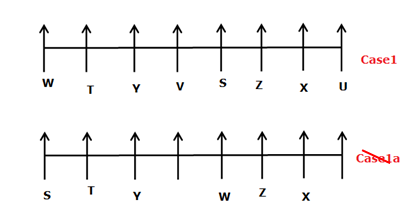Directions (6-8) :

P is taller than Q.

P > Q

Three persons are between Q and P.

P>_>_>_>Q>_

_>P>_>_>_>Q

The number of persons taller than S is the same as shorter than R.

P >_>S/R>S/R > Q >_

_>P > S/R > S/R >_>Q

U is neither the tallest nor the shortest one.

U is taller than S.

P > U(165) > S/R > S/R > Q > T

U+S=325

S=325-165=160

S+R=315

R=315-160=155cm

563812479354

531495

134559